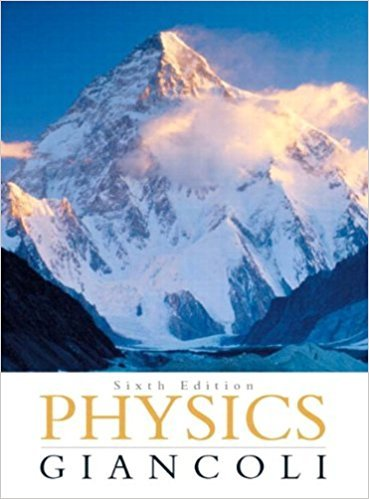×
×

# Answer: A jet plane traveling 1890 km/h (525 m/s) pullsISBN: 9780321569837 99

## Solution for problem 2P Chapter 5

Physics: Principles with Applications | 6th Edition

• Textbook Solutions
• 2901 Step-by-step solutions solved by professors and subject experts
• Get 24/7 help from StudySoup virtual teaching assistantsPhysics: Principles with Applications | 6th Edition

4 5 1 300 Reviews
26
2
Problem 2P

A jet plane traveling 1890 km/h (525 m/s) pulls out of a dive by moving in an arc of radius 6.00 km. What is the plane’s acceleration in g’s?

Step-by-Step Solution:

Solution 2P

Step 1 of 3</p>

Here we have to calculate the acceleration of the plane in terms of acceleration due to gravityon Earth surface. The plane is performing circular motion, so here we need to calculate the centripetal acceleration.

Given data,

Linear speed of plane,Radius ,UsingAcceleration due to gravity,To find,

Centripetal acceleration,Step 2 of 3</p>

For an object performing uniform circular motion, the centripetal force acts on it. Due to which it has the centripetal acceleration acts on it and is given by the rate of change of tangential velocity as in figure belowWhen an object moves in a circle with uniform speed, it has a centripetal acceleration , directed toward the center. This centripetal acceleration is directed along a radius so it may also be called the radial acceleration.

The expression for the centripetal acceleration() ,=Where v are the linear velocity of the object and r is the radius.

Step 3 of 3

##### ISBN: 9780321569837

Unlock Textbook Solution